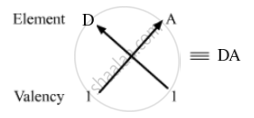# The Electrons in the Atoms of Four Elements A, B, C and D Are Distributed in Three Shells Having 1, 3, 5 and 7 Electrons in the Outermost Shell Respectively. - Science

The electrons in the atoms of four elements A, B, C and D are distributed in three shells having 1, 3, 5 and 7 electrons in the outermost shell respectively. State the period in which these elements can be placed in  the modern periodic table. Write the electronic configuration of the atoms of A and D and the molecular formula of the compound formed when A and D combine.

#### Solution

 Elements Valence electrons Period A 1 3 B 3 3 C 5 3 D 7 3

Electronic configuration of A: 1s2 2s2 2p6 3s1

Electronic configuration of D: 1s2 2s2 2p6 3s2 3p5

Molecular formula of the compound formed with A and D:

Atomic number of A = 11

Electronic configuration A = 2,8,1

Number of valence electrons of A = 1

Valency of A = 1

Atomic number of D = 17

Electronic configuration D = 2,8,7

Number of valence electrons of D = 7

Valency of D = 8-7 = 1So, the formula of the compound formed when elements A and D combine is AD.

Concept: Periodic Properties
Is there an error in this question or solution?